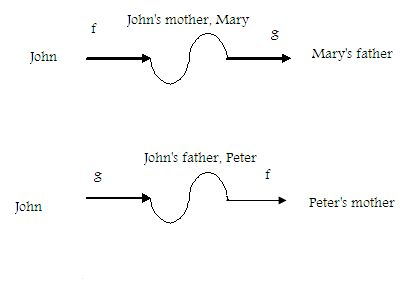kwizNET Subscribers, please login to turn off the Ads!
 Email us to get an instant 20% discount on highly effective K-12 Math & English kwizNET Programs!

#### Online Quiz (WorksheetABCD)

Questions Per Quiz = 2 4 6 8 10

### High School Mathematics - 24.6 Composition of Functions

 The basic idea of functions is that kind of a chain reaction in which the functions occur one after the other. Let f be a function whic associates with each person that person's mother and g be a function which associates with each person that person's father. Then f = {(x,y): x is a person and y is the mother of x} and g = {(x,y): x is a person and y is the father of x} We compose f with g by "applying" f and then g.We shall use the symbol "g(f)" to denote the composition of f with g. f(John) = John's mother Mary and g (Mary) = Mary's mother Thus g(Mary) = g(f(John)) and in the sense the "g" comes before "f". Therefore, we say that g(f)(John) = g(f(John)) = John's maternal grandfather. Similarly, f(g(Hari)) = f(Peter) = Peter's mother = John's paternal grandmother. Definition: Given two functions, f and g, the function x -> g(f(x)) is called a composite of f and g and is denoted by gf. The domain of gf is the set of all elements x in the domain of f for which f(x) is in the domain of g. The operation of formng a composite of two functions is called composition. The function g(f(x)) is also denoted by (gof)(x) and is read as 'g circle f'. Notice that the order of the symbolsis the same as in g(f(x), i.e, f is applied first, then g. The composition of functions is associative. Example: Given that f: x -> 3x-2 and g:x ->x5 for all x € R, find (gf)(x) (gf)(x) = g(f(x) = g(3x-2) = (3x-2)5 Solution: (3x-2)5 Directions: Find the composite functions of the following.
 Q 1: If f(x) = (x+1)/(x-1), x not equal to 1, find (fofofof)(x)1xx-1 Q 2: If f: x -> 2x, g: x -> x2 and h: x -> x +1. Find the formula for ho(gof)4x4x+14x2+1 Q 3: Given that f: x -> 3x-2 and g:x ->x5 for all x € R, find f(g(x)+3)(3x-2)53x5+79x-8 Q 4: Given that f: x -> 3x-2 and g:x ->x5 for all x € R, find f(g(x) -f(x)) 3x5-9x+4(3x-2)5(2x-3)5 Q 5: Given f(x) = 3 , g(x) = 5y, find f(x).g(x).5y3y15y Q 6: If f: R -> R, g: R -> R are functions defined by f(x) = 3x -2 , g(x) - x2 + 1, find (gof-1)(2)1/225/94/3 Q 7: Given that f: x -> 3x-2 and g:x ->x5 for all x € R, find (ff)(x).9x-83x-2(3x-2)5 Q 8: If z = g(y) = 3y2 - 2y + 1 and y = f(x) = 4x+7, find the composite function gof.(4x+5)24x+748x2 + 160x + 134 Question 9: This question is available to subscribers only! Question 10: This question is available to subscribers only!

#### Subscription to kwizNET Learning System offers the following benefits:

• Unrestricted access to grade appropriate lessons, quizzes, & printable worksheets
• Instant scoring of online quizzes
• Progress tracking and award certificates to keep your student motivated
• Unlimited practice with auto-generated 'WIZ MATH' quizzes
• Child-friendly website with no advertisements
• Choice of Math, English, Science, & Social Studies Curriculums
• Excellent value for K-12 and ACT, SAT, & TOEFL Test Preparation
• Get discount offers by sending an email to discounts@kwiznet.com

 Quiz Timer